Hypothesis Testing with Two Samples

# 54 Matched or Paired Samples

In most cases of economic or business data we have little or no control over the process of how the data are gathered. In this sense the data are not the result of a planned controlled experiment. In some cases, however, we can develop data that are part of a controlled experiment. This situation occurs frequently in quality control situations. Imagine that the production rates of two machines built to the same design, but at different manufacturing plants, are being tested for differences in some production metric such as speed of output or meeting some production specification such as strength of the product. The test is the same in format to what we have been testing, but here we can have matched pairs for which we can test if differences exist. Each observation has its matched pair against which differences are calculated. First, the differences in the metric to be tested between the two lists of observations must be calculated, and this is typically labeled with the letter “d.” Then, the average of these matched differences,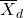is calculated as is its standard deviation, Sd. We expect that the standard deviation of the differences of the matched pairs will be smaller than unmatched pairs because presumably fewer differences should exist because of the correlation between the two groups.

When using a hypothesis test for matched or paired samples, the following characteristics may be present:

1. Simple random sampling is used.
2. Sample sizes are often small.
3. Two measurements (samples) are drawn from the same pair of individuals or objects.
4. Differences are calculated from the matched or paired samples.
5. The differences form the sample that is used for the hypothesis test.
6. Either the matched pairs have differences that come from a population that is normal or the number of differences is sufficiently large so that distribution of the sample mean of differences is approximately normal.

In a hypothesis test for matched or paired samples, subjects are matched in pairs and differences are calculated. The differences are the data. The population mean for the differences, μd, is then tested using a Student’s-t test for a single population mean with n – 1 degrees of freedom, where n is the number of differences, that is, the number of pairs not the number of observations.

The null and alternative hypotheses for this test are: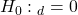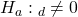The test statistic is: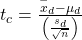A company has developed a training program for its entering employees because they have become concerned with the results of the six-month employee review. They hope that the training program can result in better six-month reviews. Each trainee constitutes a “pair”, the entering score the employee received when first entering the firm and the score given at the six-month review. The difference in the two scores were calculated for each employee and the means for before and after the training program was calculated. The sample mean before the training program was 20.4 and the sample mean after the training program was 23.9. The standard deviation of the differences in the two scores across the 20 employees was 3.8 points. Test at the 10% significance level the null hypothesis that the two population means are equal against the alternative that the training program helps improve the employees’ scores.

The first step is to identify this as a two sample case: before the training and after the training. This differentiates this problem from simple one sample issues. Second, we determine that the two samples are “paired.” Each observation in the first sample has a paired observation in the second sample. This information tells us that the null and alternative hypotheses should be: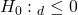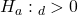This form reflects the implied claim that the training course improves scores; the test is one-tailed and the claim is in the alternative hypothesis. Because the experiment was conducted as a matched paired sample rather than simply taking scores from people who took the training course those who didn’t, we use the matched pair test statistic: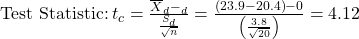In order to solve this equation, the individual scores, pre-training course and post-training course need to be used to calculate the individual differences. These scores are then averaged and the average difference is calculated: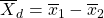From these differences we can calculate the standard deviation across the individual differences: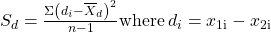We can now compare the calculated value of the test statistic, 4.12, with the critical value. The critical value is a Student’s t with degrees of freedom equal to the number of pairs, not observations, minus 1. In this case 20 pairs and at 90% confidence level ta/2 = ±1.729 at df = 20 – 1 = 19. The calculated test statistic is most certainly in the tail of the distribution and thus we cannot accept the null hypothesis that there is no difference from the training program. Evidence seems indicate that the training aids employees in gaining higher scores.

A study was conducted to investigate the effectiveness of hypnotism in reducing pain. Results for randomly selected subjects are shown in (Figure). A lower score indicates less pain. The “before” value is matched to an “after” value and the differences are calculated. Are the sensory measurements, on average, lower after hypnotism? Test at a 5% significance level.

Subject: A B C D E F G H
Before 6.6 6.5 9.0 10.3 11.3 8.1 6.3 11.6
After 6.8 2.4 7.4 8.5 8.1 6.1 3.4 2.0

Corresponding “before” and “after” values form matched pairs. (Calculate “after” – “before.”)

After data Before data Difference
6.8 6.6 0.2
2.4 6.5 -4.1
7.4 9 -1.6
8.5 10.3 -1.8
8.1 11.3 -3.2
6.1 8.1 -2
3.4 6.3 -2.9
2 11.6 -9.6

The data for the test are the differences: {0.2, –4.1, –1.6, –1.8, –3.2, –2, –2.9, –9.6}

The sample mean and sample standard deviation of the differences are: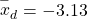and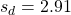Verify these values.

Let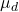be the population mean for the differences. We use the subscript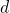to denote “differences.”

Random variable: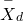= the mean difference of the sensory measurements

H0: μd ≥ 0

The null hypothesis is zero or positive, meaning that there is the same or more pain felt after hypnotism. That means the subject shows no improvement. μd is the population mean of the differences.)

Ha: μd < 0

The alternative hypothesis is negative, meaning there is less pain felt after hypnotism. That means the subject shows improvement. The score should be lower after hypnotism, so the difference ought to be negative to indicate improvement.

Distribution for the test: The distribution is a Student’s t with df = n – 1 = 8 – 1 = 7. Use t7. (Notice that the test is for a single population mean.)

Calculate the test statistic and look up the critical value using the Student’s-t distribution: The calculated value of the test statistic is 3.06 and the critical value of the t distribution with 7 degrees of freedom at the 5% level of confidence is 1.895 with a one-tailed test.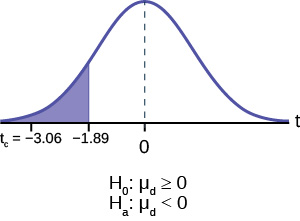is the random variable for the differences.

The sample mean and sample standard deviation of the differences are: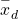= –3.13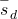= 2.91

Compare the critical value for alpha against the calculated test statistic.

The conclusion from using the comparison of the calculated test statistic and the critical value will gives us the result. In this question the calculated test statistic is 3.06 and the critical value is 1.895. The test statistic is clearly in the tail and thus we cannot accept the null hypotheses that there is no difference between the two situations, hypnotized and not hypnotized.

Make a decision: Cannot accept the null hypothesis, H0. This means that μd < 0 and there is a statistically significant improvement.

Conclusion: At a 5% level of significance, from the sample data, there is sufficient evidence to conclude that the sensory measurements, on average, are lower after hypnotism. Hypnotism appears to be effective in reducing pain.

A college football coach was interested in whether the college’s strength development class increased his players’ maximum lift (in pounds) on the bench press exercise. He asked four of his players to participate in a study. The amount of weight they could each lift was recorded before they took the strength development class. After completing the class, the amount of weight they could each lift was again measured. The data are as follows:

Weight (in pounds) Player 1 Player 2 Player 3 Player 4
Amount of weight lifted prior to the class 205 241 338 368
Amount of weight lifted after the class 295 252 330 360

The coach wants to know if the strength development class makes his players stronger, on average.
Record the differences data. Calculate the differences by subtracting the amount of weight lifted prior to the class from the weight lifted after completing the class. The data for the differences are: {90, 11, -8, -8}.= 21.3, sd = 46.7

Using the difference data, this becomes a test of a single mean.

Define the random variable:mean difference in the maximum lift per player.

The distribution for the hypothesis test is a student’s t with 3 degrees of freedom.

H0: μd ≤ 0, Ha: μd > 0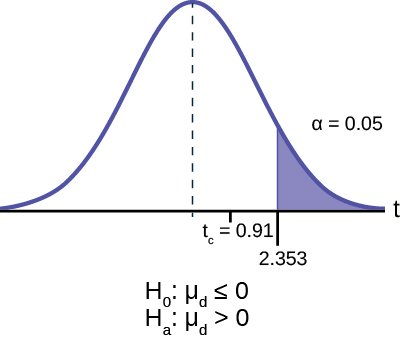Calculate the test statistic look up the critical value: Critical value of the test statistic is 0.91. The critical value of the student’s t at 5% level of significance and 3 degrees of freedom is 2.353.

Decision: If the level of significance is 5%, we cannot reject the null hypothesis, because the calculated value of the test statistic is not in the tail.

What is the conclusion?

At a 5% level of significance, from the sample data, there is not sufficient evidence to conclude that the strength development class helped to make the players stronger, on average.

### Chapter Review

A hypothesis test for matched or paired samples (t-test) has these characteristics:

• Test the differences by subtracting one measurement from the other measurement
• Random Variable:= mean of the differences
• Distribution: Student’s-t distribution with n – 1 degrees of freedom
• If the number of differences is small (less than 30), the differences must follow a normal distribution.
• Two samples are drawn from the same set of objects.
• Samples are dependent.

### Formula Review

Test Statistic (t-score): tc =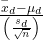where:is the mean of the sample differences. μd is the mean of the population differences. sd is the sample standard deviation of the differences. n is the sample size.

Use the following information to answer the next five exercises. A study was conducted to test the effectiveness of a software patch in reducing system failures over a six-month period. Results for randomly selected installations are shown in (Figure). The “before” value is matched to an “after” value, and the differences are calculated. The differences have a normal distribution. Test at the 1% significance level.

Installation A B C D E F G H
Before 3 6 4 2 5 8 2 6
After 1 5 2 0 1 0 2 2

What is the random variable?

the mean difference of the system failures

State the null and alternative hypotheses.

What conclusion can you draw about the software patch?

With a p-value 0.0067, we can cannot accept the null hypothesis. There is enough evidence to support that the software patch is effective in reducing the number of system failures.

Use the following information to answer next five exercises. A study was conducted to test the effectiveness of a juggling class. Before the class started, six subjects juggled as many balls as they could at once. After the class, the same six subjects juggled as many balls as they could. The differences in the number of balls are calculated. The differences have a normal distribution. Test at the 1% significance level.

Subject A B C D E F
Before 3 4 3 2 4 5
After 4 5 6 4 5 7

State the null and alternative hypotheses.

What is the sample mean difference?

What conclusion can you draw about the juggling class?

Use the following information to answer the next five exercises. A doctor wants to know if a blood pressure medication is effective. Six subjects have their blood pressures recorded. After twelve weeks on the medication, the same six subjects have their blood pressure recorded again. For this test, only systolic pressure is of concern. Test at the 1% significance level.

Patient A B C D E F
Before 161 162 165 162 166 171
After 158 159 166 160 167 169

State the null and alternative hypotheses.

H0: μd ≥ 0

Ha: μd < 0

What is the test statistic?

What is the sample mean difference?

What is the conclusion?

We decline to reject the null hypothesis. There is not sufficient evidence to support that the medication is effective.

### Homework

Ten individuals went on a low–fat diet for 12 weeks to lower their cholesterol. The data are recorded in (Figure). Do you think that their cholesterol levels were significantly lowered?

Starting cholesterol level Ending cholesterol level
140 140
220 230
110 120
240 220
200 190
180 150
190 200
360 300
280 300
260 240

p-value = 0.1494

At the 5% significance level, there is insufficient evidence to conclude that the medication lowered cholesterol levels after 12 weeks.

Use the following information to answer the next two exercises. A new AIDS prevention drug was tried on a group of 224 HIV positive patients. Forty-five patients developed AIDS after four years. In a control group of 224 HIV positive patients, 68 developed AIDS after four years. We want to test whether the method of treatment reduces the proportion of patients that develop AIDS after four years or if the proportions of the treated group and the untreated group stay the same.

Let the subscript t = treated patient and ut = untreated patient.

The appropriate hypotheses are:

1. H0: pt < put and Ha: ptput
2. H0: ptput and Ha: pt > put
3. H0: pt = put and Ha: ptput
4. H0: pt = put and Ha: pt < put

Use the following information to answer the next two exercises. An experiment is conducted to show that blood pressure can be consciously reduced in people trained in a “biofeedback exercise program.” Six subjects were randomly selected and blood pressure measurements were recorded before and after the training. The difference between blood pressures was calculated (after – before) producing the following results:= −10.2 sd = 8.4. Using the data, test the hypothesis that the blood pressure has decreased after the training.

The distribution for the test is:

1. t5
2. t6
3. N(−10.2, 8.4)
4. N(−10.2,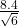)

A golf instructor is interested in determining if her new technique for improving players’ golf scores is effective. She takes four new students. She records their 18-hole scores before learning the technique and then after having taken her class. She conducts a hypothesis test. The data are as follows.

Player 1 Player 2 Player 3 Player 4
Mean score before class 83 78 93 87
Mean score after class 80 80 86 86

The correct decision is:

1. Reject H0.
2. Do not reject the H0.

A local cancer support group believes that the estimate for new female breast cancer cases in the south is higher in 2013 than in 2012. The group compared the estimates of new female breast cancer cases by southern state in 2012 and in 2013. The results are in (Figure).

Southern states 2012 2013
Alabama 3,450 3,720
Arkansas 2,150 2,280
Florida 15,540 15,710
Georgia 6,970 7,310
Kentucky 3,160 3,300
Louisiana 3,320 3,630
Mississippi 1,990 2,080
North Carolina 7,090 7,430
Oklahoma 2,630 2,690
South Carolina 3,570 3,580
Tennessee 4,680 5,070
Texas 15,050 14,980
Virginia 6,190 6,280

Test: two matched pairs or paired samples (t-test)

Random variable:Distribution: t12

H0: μd = 0 Ha: μd > 0

The mean of the differences of new female breast cancer cases in the south between 2013 and 2012 is greater than zero. The estimate for new female breast cancer cases in the south is higher in 2013 than in 2012.

Graph: right-tailed

p-value: 0.0004

Decision: Cannot accept H0

Conclusion: At the 5% level of significance, from the sample data, there is sufficient evidence to conclude that there was a higher estimate of new female breast cancer cases in 2013 than in 2012.

A traveler wanted to know if the prices of hotels are different in the ten cities that he visits the most often. The list of the cities with the corresponding hotel prices for his two favorite hotel chains is in (Figure). Test at the 1% level of significance.

Cities Hyatt Regency prices in dollars Hilton prices in dollars
Atlanta 107 169
Boston 358 289
Chicago 209 299
Dallas 209 198
Denver 167 169
Indianapolis 179 214
Los Angeles 179 169
New York City 625 459
Washington, DC 245 239

A politician asked his staff to determine whether the underemployment rate in the northeast decreased from 2011 to 2012. The results are in (Figure).

Northeastern states 2011 2012
Connecticut 17.3 16.4
Delaware 17.4 13.7
Maine 19.3 16.1
Maryland 16.0 15.5
Massachusetts 17.6 18.2
New Hampshire 15.4 13.5
New Jersey 19.2 18.7
New York 18.5 18.7
Ohio 18.2 18.8
Pennsylvania 16.5 16.9
Rhode Island 20.7 22.4
Vermont 14.7 12.3
West Virginia 15.5 17.3

Test: matched or paired samples (t-test)

Difference data: {–0.9, –3.7, –3.2, –0.5, 0.6, –1.9, –0.5, 0.2, 0.6, 0.4, 1.7, –2.4, 1.8}

Random Variable:Distribution: H0: μd = 0 Ha: μd < 0

The mean of the differences of the rate of underemployment in the northeastern states between 2012 and 2011 is less than zero. The underemployment rate went down from 2011 to 2012.

Graph: left-tailed.

Decision: Cannot reject H0.

Conclusion: At the 5% level of significance, from the sample data, there is not sufficient evidence to conclude that there was a decrease in the underemployment rates of the northeastern states from 2011 to 2012.

### Bringing It Together

Use the following information to answer the next ten exercises. indicate which of the following choices best identifies the hypothesis test.

1. independent group means, population standard deviations and/or variances known
2. independent group means, population standard deviations and/or variances unknown
3. matched or paired samples
4. single mean
5. two proportions
6. single proportion

A powder diet is tested on 49 people, and a liquid diet is tested on 36 different people. The population standard deviations are two pounds and three pounds, respectively. Of interest is whether the liquid diet yields a higher mean weight loss than the powder diet.

A new chocolate bar is taste-tested on consumers. Of interest is whether the proportion of children who like the new chocolate bar is greater than the proportion of adults who like it.

e

The mean number of English courses taken in a two–year time period by male and female college students is believed to be about the same. An experiment is conducted and data are collected from nine males and 16 females.

A football league reported that the mean number of touchdowns per game was five. A study is done to determine if the mean number of touchdowns has decreased.

d

A study is done to determine if students in the California state university system take longer to graduate than students enrolled in private universities. One hundred students from both the California state university system and private universities are surveyed. From years of research, it is known that the population standard deviations are 1.5811 years and one year, respectively.

According to a YWCA Rape Crisis Center newsletter, 75% of rape victims know their attackers. A study is done to verify this.

f

According to a recent study, U.S. companies have a mean maternity-leave of six weeks.

A recent drug survey showed an increase in use of drugs and alcohol among local high school students as compared to the national percent. Suppose that a survey of 100 local youths and 100 national youths is conducted to see if the proportion of drug and alcohol use is higher locally than nationally.

e

A new SAT study course is tested on 12 individuals. Pre-course and post-course scores are recorded. Of interest is the mean increase in SAT scores. The following data are collected:

Pre-course score Post-course score
1 300
960 920
1010 1100
840 880
1100 1070
1250 1320
860 860
1330 1370
790 770
990 1040
1110 1200
740 850

University of Michigan researchers reported in the Journal of the National Cancer Institute that quitting smoking is especially beneficial for those under age 49. In this American Cancer Society study, the risk (probability) of dying of lung cancer was about the same as for those who had never smoked.

f

Lesley E. Tan investigated the relationship between left-handedness vs. right-handedness and motor competence in preschool children. Random samples of 41 left-handed preschool children and 41 right-handed preschool children were given several tests of motor skills to determine if there is evidence of a difference between the children based on this experiment. The experiment produced the means and standard deviations shown (Figure). Determine the appropriate test and best distribution to use for that test.

 Left-handed Right-handed Sample size 41 41 Sample mean 97.5 98.1 Sample standard deviation 17.5 19.2
1. Two independent means, normal distribution
2. Two independent means, Student’s-t distribution
3. Matched or paired samples, Student’s-t distribution
4. Two population proportions, normal distribution

A golf instructor is interested in determining if her new technique for improving players’ golf scores is effective. She takes four (4) new students. She records their 18-hole scores before learning the technique and then after having taken her class. She conducts a hypothesis test. The data are as (Figure).

Player 1 Player 2 Player 3 Player 4
Mean score before class 83 78 93 87
Mean score after class 80 80 86 86

This is:

1. a test of two independent means.
2. a test of two proportions.
3. a test of a single mean.
4. a test of a single proportion.

a